# Analytics Calculations: Match+Index I: Top Categories by Store

Moderator, Lightspeed Staff Posts: 66 moderator
Match+Index is a powerful combination of functions that finds where something is on your report and then returns the result to where you want it. While this combination has many sophisticated applications, we can start with a simple model.

Let's say for example, that we are still interested in reporting on the top categories of sales by store, but we are using a pivot on store...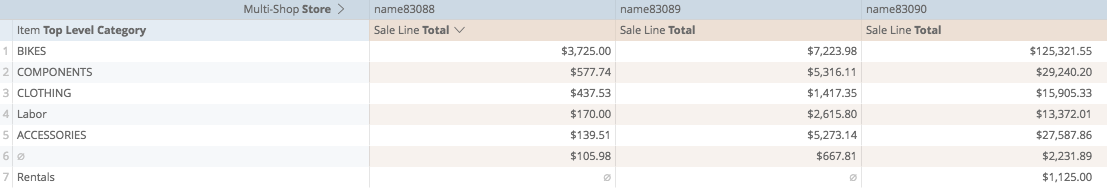Using the "Large" function from our math-based functions, we can identify the largest value from a column.

The "Large" function requires two arguments: which column we're looking at and which position of large we're looking for, (is it the 1st largest, 2nd largest, etc...)

For our first argument, we'll ask the calculation to look at the sale-line total...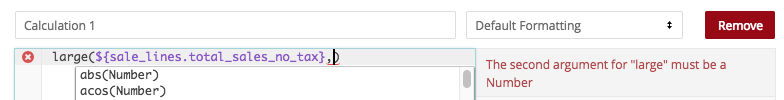and for the second argument, we'll ask the calculation to look at the row number. This way, the highest value will be on top, the second-highest value will be in the second row, the third-highest value will be in the third row, and so on...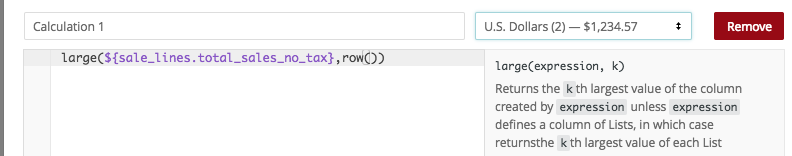So my first calculation here looks like
large(\${sale_lines.total_sales_no_tax},row())

If I have prepared my calculation properly, I will a column ranking the values from highest to lowest...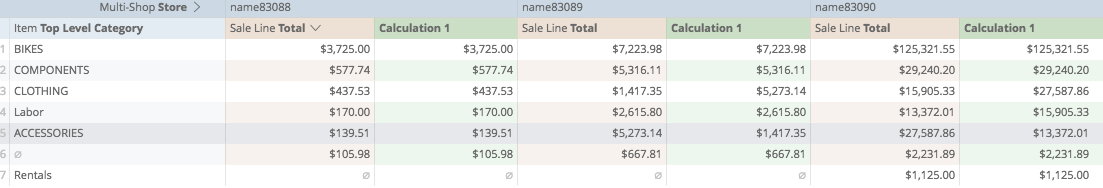But it's only showing me the value that is highest, not the category that is highest...

So the next thing I want to do, is report on which row each value is coming from. For this we can use the "Match" function from our table-functions.

The Match function needs two arguments: which value we're looking for, and the column in which we are looking for it.

Following our best-practices, let's open up a new calculation, and start building it with brackets...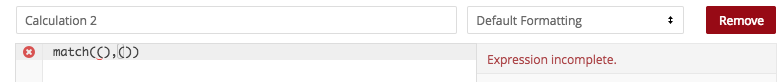then we'll copy our first calculation, referencing what we're looking for...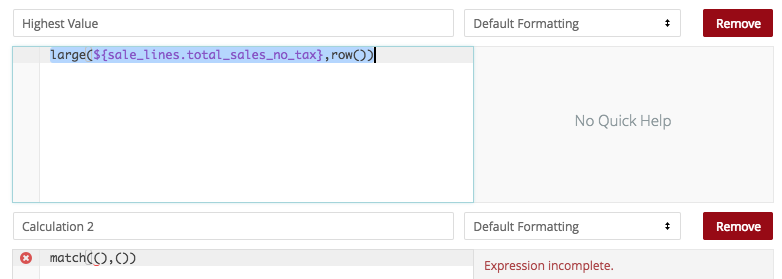...and paste it into the first set of brackets...next, we'll add the second argument, where we are looking for it; the sales totals...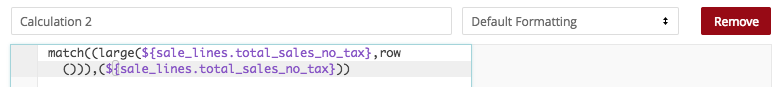now my calculation looks like:

match((large(\${sale_lines.total_sales_no_tax},row())),(\${sale_lines.total_sales_no_tax}))

and if I have prepared it properly, when I save...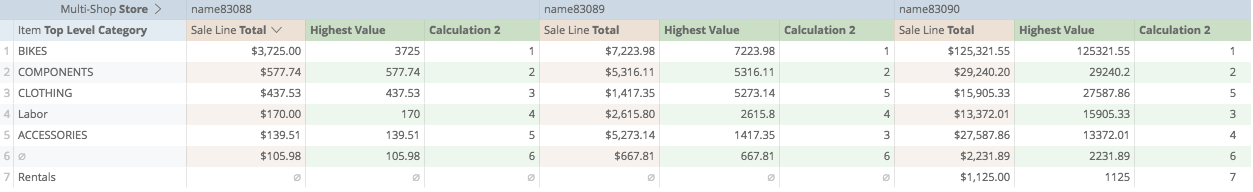We now get a second column showing which row the value can be found in...

In the first store, this is unremarkable because the default sorting is from highest to lowest...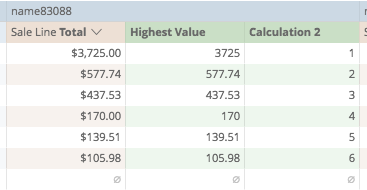but in the other stores, we see that the referenced high categories do not line up with their respective rows...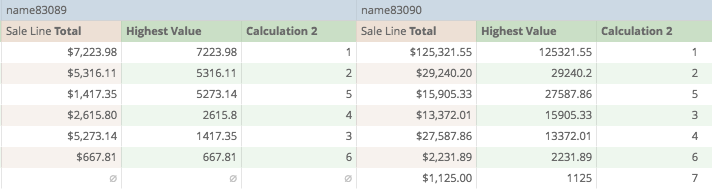Now, keeping up with our best practices, let's save a working version of this report...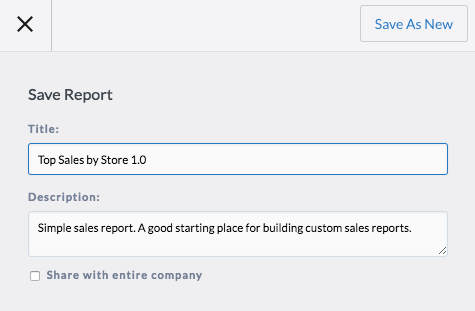Now on our report, we don't just want to know what row the value is on, we want to know what the actual category is.

If we know which row the value is on, we can ask Analytics to find the category from that row...

From our table-functions, we can use the Index function to do this. The Index function needs two arguments, which column we're looking at, and which row we're asking it to look in...

We'll create a new calculation, and again, we'll start it with brackets...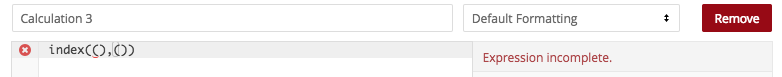...and we'll start by asking it to look at the column of Categories...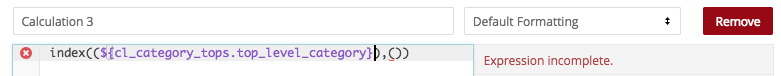...then we copy the calculation from the field above...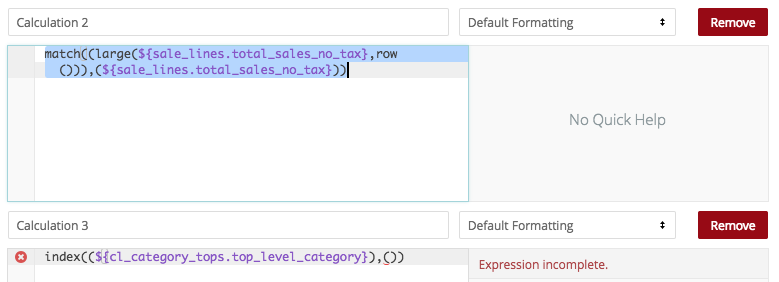...and paste it into our final calculation below...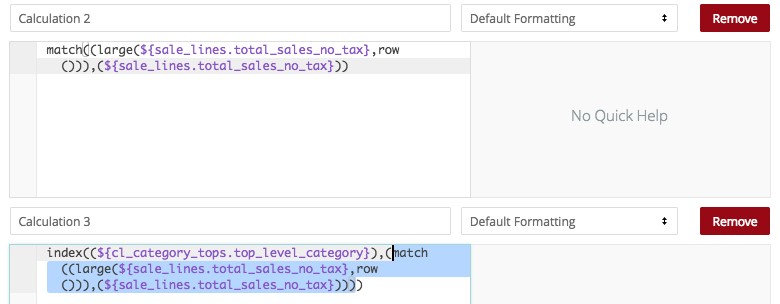So our final calculation now looks like:

index((\${cl_category_tops.top_level_category}),(match((large(\${sale_lines.total_sales_no_tax},row())),(\${sale_lines.total_sales_no_tax}))))

and if I have prepared it properly, now when I save...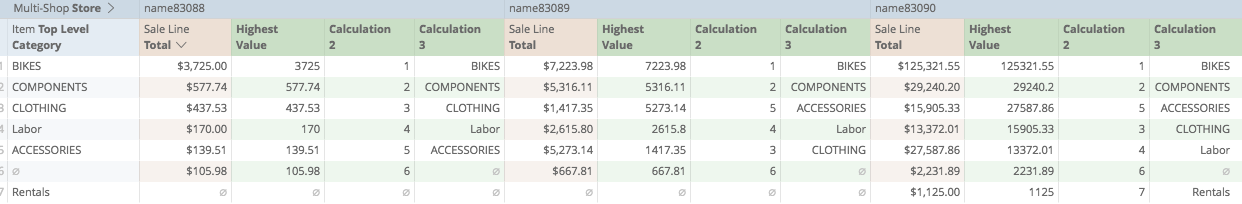We get a final calculation showing us the label of the category corresponding to the value amount!

Again, let's save this as a new version of the report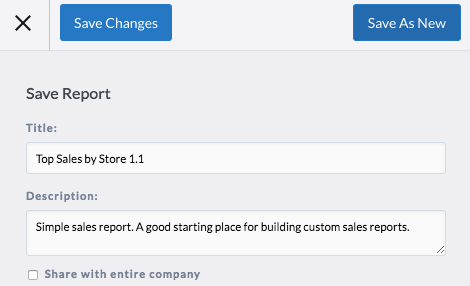Finally, let's do some cosmetics to make it meaningful.

First, let's hide the Dimension "Top Level Category" from visualization, we'll be using the Calculation to show us the Category labels...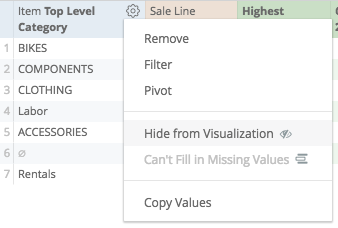Next, let's hide the "Sale Line Total" from visualization, because we will also be using the Calculation to display these numbers...Then, we can remove Calculation 2, showing us which row the values are on. This is not necessary to display, and is implicit in the final calculation, so it doesn't need to be on the report anymore...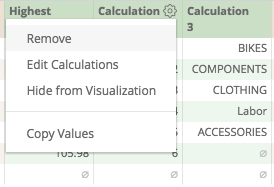Our visualization is looking a bit better...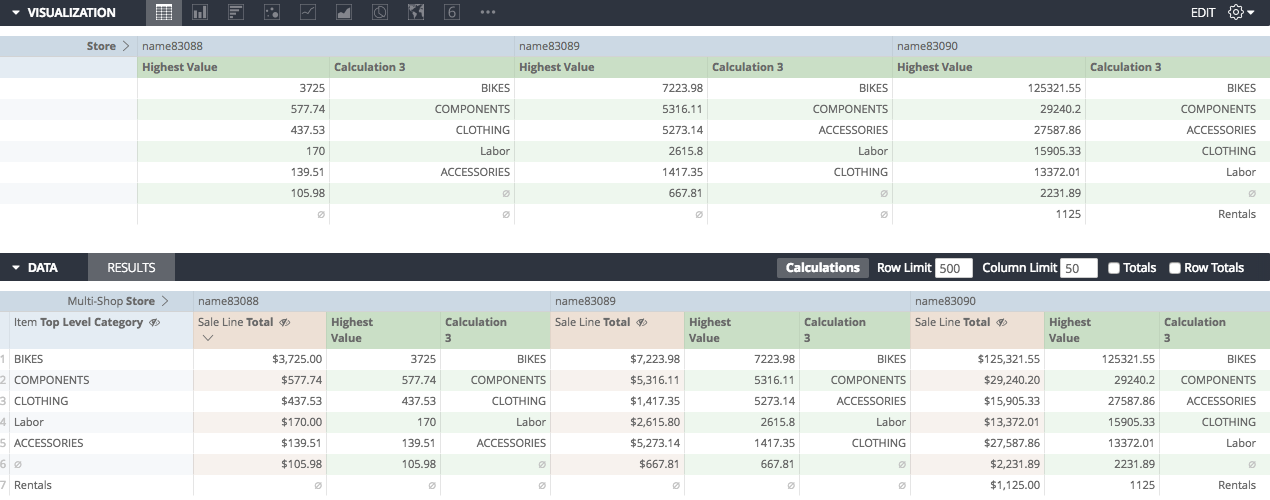Let's also change the order of Calculations, so that the Category shows up first, and the Total shows up second.

The best way to do this is start a new calculation, and then copy+past the first one...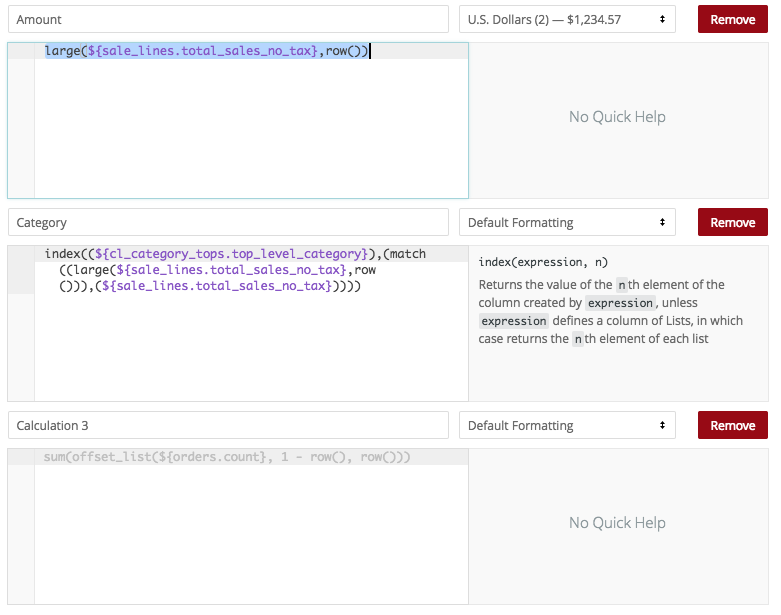into the last...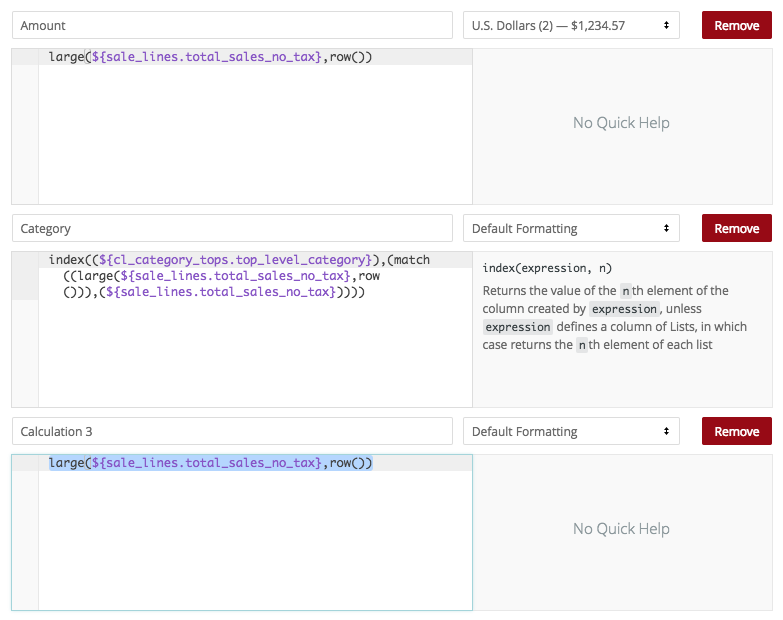...then remove the first calculation...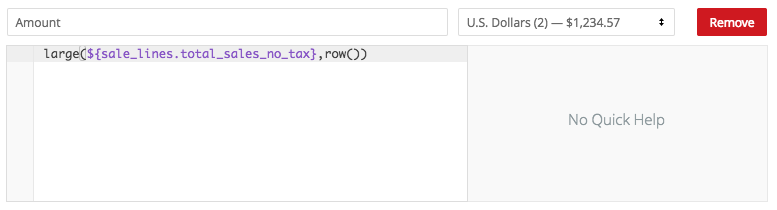...so that when we finally save...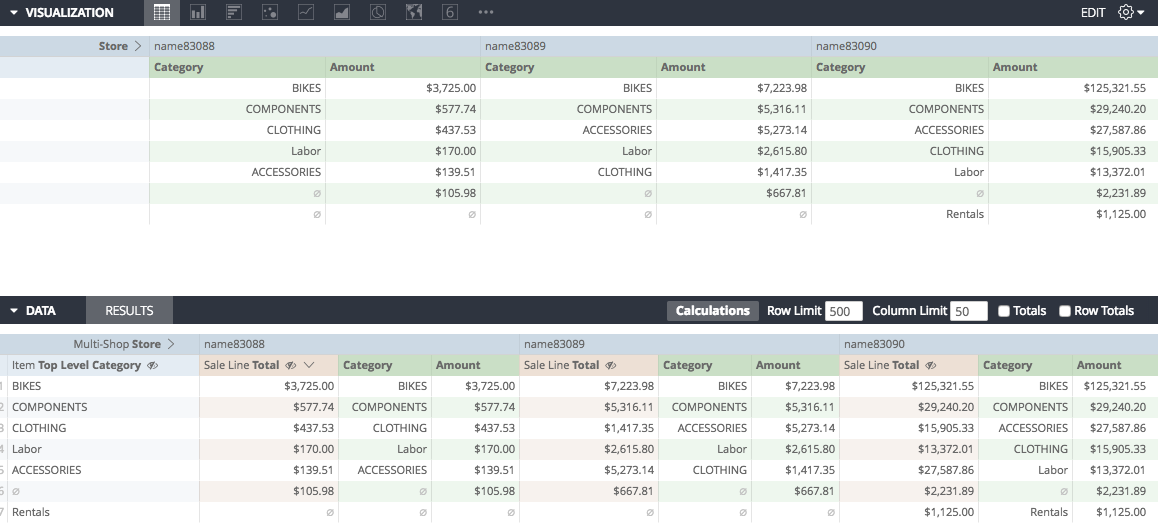we have a meaningful report showing us top levels of categories and totals by store!

*Note this approach to match+index works best when no duplicate values are anticipated. Using it to illustrate quantity of products sold in a small time-frame may create unanticipated results if multiple products sold the same quantity. In this case, more calculationing may be required

Calculations Home
Joseph McKeown
Lightspeed Analytics and reporting consultant
Lightspeed HQ